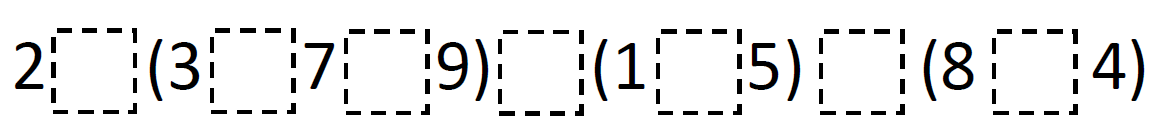Home > Grade 5 > Balanced Equations 2

# Balanced Equations 2

Directions: Use the operation symbols (+, -, x, ÷) and equal sign (=) to make a true equation. Operation symbols may be used more than once.
What is the least value for each part of the equation? What is the greatest value for each part of the equation?
Can you complete the equation with at least one of each symbol?### Hint

Where might you place the equals sign(s)?

Several answers are possible such as 2 x (3 + 7 – 9) x (1 + 5) = (8 + 4)
2 x (3 + 7 + 9) = (1 + 5) + (8 x 4)

Using each symbol at least once, here is one solution: 2=(3×7-9)/(1+5)=(8/4)

Source: Joshua Nelson and Renee Owen

## Subtracting Decimals 2

Directions: Using the digits 1-9, at most once each, fill in the boxes to make …

1.2.